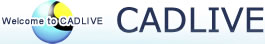### CADLIVE SIMULATOR(Last update August 23, 2010)

* Use Internet Explorer. The simulator does not support the other applications.
* If there is anything wrong with your screen, take care of the encode of your browser.

### 2. How to learn CADLIVE

#### 2.1 Instruction of CADLIVE Simulator

The important thing is to learn how to convert regulator-reaction equations to a mathematical model. Once regulator-reaction equations are described with correct mathematical conversion tags, it is easy to simulate the generated mathematical model, because it is written in a similar format as employed ordinary programming language. Users set the initial molecular concentrations and kinetic parameters in the model, and simulate it. Since the mathematical conversion rules (parsedae module) have been originally proposed, most scientists may not be familiar with these conversion rules. However, users will understand for themselves, because the rules are designed plainly. We show an efficient way to learn CADLIVE.

#### 2.2 How to learn CADLIVE

We recommend users to learn CADLIVE by using the presented examples. We believe that the presented examples are satisfactory for learning how to use the CADLIVE Simulator. However, it may be hard for some scientists. Thus, we have software demonstrations in many places. If you have questions on CADLIVE, please ask us (kurata@bio.kyutech.ac.jp).

##### 1. Learn GUI editors.

Users learn how to describe a biochemical map by using the GUI editors. Detailed instruction is described on the web page and in our paper (Kurata, H., N. Matoba, and N. Shimizu. 2003. CADLIVE for constructing a large-scale biochemical network based on a simulation-directed notation and its application to yeast cell cycle. Nucleic Acids Res 31: 4071-4084.).

##### 2. Understand the overview of the Simulator

Read the overview of CADLIVE Simulator to know the overview of how to use the simulator. This instruction is very helpful for understanding the basic functions of CADLIVE. The simulator consists of:

Converting them into a mathematical model,
Setting initial values and kinetic parameters,
simulating the time course of the model.
When obtaining the steady-state values, users are able to run the steady-state analysis.
##### 3. Learn how to simulate a model

We recommend users to learn the conversion rules (parsedae module in the technical description) using the presented examples: heat shock response and nitrogen assimilation system.

3.1 Heat shock response

Users should learn three things.

i) Conversion rules

The heat shock response is a plain example to understand the definition of conversion rules, such as complex, modified, enhancer, and promoter, and to learn how to covert a map to a mathematical model according to the rules. Trial and error may be necessary for understanding the relationships between biochemical maps and mathematical models. (Dynamic simulation of DAEs using Two-Phase Partition method)

ii) How to get the initial values of DAEs

In order to solve DAEs numerically, how to obtain initial values is critical. First, simulate the CMA corresponding to the DAEs to find the values close to the steady state values. And the obtained values should be employed as the initial values of the DAEs. (Dynamic simulation of CMA)

iii) System analysis

Users learn how to carry out the steady-state analysis that characterizes the sensitivity and stability. (Steady state analysis of DAEs) After simulating DAEs or CMA for a long time, the final concentrations are set as the initial values for solving algebraic equations at the steady state.

3.2 Nitrogen assimilation system

Users lean how to handle metabolic equations and to connect them to the gene and protein layers.

##### 4. Understand optimizers

It is required to optimize a dynamic model so that it reproduces experimental behaviors. CADLIVE presents the modules for parameter searches (GAs). How to optimize a system is described in the instruction of optimizer. This module works on command lines in Linux.

##### 5. S-system analysis

S-system conversion and analysis: See the example for S-system of TCA cycle in our web site.

### 3. Detailed instructions

#### 3.1 CADLIVE Editor (published in Nucleic Acids Res. 31 4071-4084, 2003)

GUI editor (PDF file)
Text editor (PDF file)

3.2.1 Instllation (PDF file)
3.2.2 Overview of CADLIVE Simulator (PDF file)
3.3.3 Parsedae(converting regulator-reaction equations into dynamic models) (PDF file)
3.3.4 Checkdae (converting dynamic models into user functions) (PDF file)
3.3.5 Optimizer (Genetic Algorithms) (PDF file)
3.3.6 Sensitivity and stability analysis (PDF file)
3.3.7 S-system (PDF file)

### 4. Simulation Examples

"sanac.dtd" must be put in the same directory for opening the following XML files.

#### 4.1 Heat shock response system

Instruction: Dynamic simulation of CMA (pdf)
Instruction: Dynamic simulation of DAEs using Two-Phase Partition method. (pdf)
Instruction: Steady state analysis of DAEs (pdf)
(HSR_demo.xml, TPP_Param_HSR.txt, CMA_Param_HSR.txt)

#### 4.2 Nitrogen assimilation system

Instruction: Dynamic simulation of DAEs using Two-Phase Partition method. (pdf)
Instruction: Steady state analysis of DAEs (pdf)
(NFS_demo.xml, TPP_Param_NFS.txt)

Instruction: Dynamic simulation of DAEs using Two-Phase Partition method (pdf)